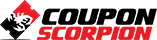# Free Math Tutorial – Numerical Root Finding in Python and MATLAB

A Hands-on Approach – Free Course

### What you’ll learn

• Theory of Bisection, Secant and Newton-Raphson Methods
• Python Implementation of 3 Root Finding Methods
• MATLAB Implementation of 3 Root Finding Methods

### Requirements

• Basic Mathematics
• Python Programming
• MATLAB Programming

### Description

This series of video tutorials covers the numerical methods for Root Finding (Solving Algebraic Equations) from theory to implementation. In this course, three methods are reviewed and implemented using Python and MATLAB from scratch.

At first, two interval-based methods, namely Bisection method and Secant method, are reviewed and implemented. Then, a point-based method which is known as Newton’s method for root finding, a.k.a. NewtonRaphson method, is reviewed and implemented. This course is instructed by Dr. Mostapha Kalami Heris, who has years of practical work and active teaching in the field of programming, mathematics, control engineering and computational intelligence.

By the end of this course you will be able to know about the fundamental theory of this root finding methods and implementing them using Python and MATLAB programming languages.

Author(s): Yarpiz Team, Mostapha Kalami Heris

Deal Score+7
FREE 0